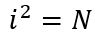# Problem 50132. Number Puzzle - 033

Give an example of a number i where i>1000 that satisfies the following conditions:and N does not have the digit "3" in it but the sum of the digits of N is equal to 3^2 or 9.

### Solution Stats

68.75% Correct | 31.25% Incorrect
Last Solution submitted on Aug 24, 2023

### Community Treasure Hunt

Find the treasures in MATLAB Central and discover how the community can help you!

Start Hunting!